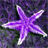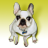# Two iterators in modelbuilder

16198
14
08-15-2012 11:18 PMNew Contributor
Hi.
I am working on a project were I need to combine several files. It can be illustrated like this:
I need to open File_1 and do a calculation within Mask_A. Then File_2 within Mask_B and so forth. Both the files and masks are stored in separate folders. Is this possible to do in ModelBuilder? I guess I have to use Python, so maybe one of you have an input to that?
It is easy to do in ModelBuilder if I want to use the same mask for all Files, but not if I want to change mask for each file.
Tags (3)
14 RepliesbyEsri Contributor
Hi Andreas123
There is no possibility to use two iterators within one model. But if there is a logic to match the file_name to the mask_name, you can use the model-only tool "Calculate Value" to map the right mask to the current file.
JochenNew Contributor III

Can you elaborate on how you would use the Calculate Value tool to match a file_name to a mask_name? I have raster that are named something like ID_distance and bufffers that are also named ID_distance and I need to run zonal statistics for each raster (about 1300 rasters) in each corresponding buffer. Any ideas?

ThanksbyEsri Notable Contributor
You can imbed one model within another, so that you can use more than one iterator.  Setting preconditions will make sure that both iterators run before you do any further processing.  Here's a resource that will help get you started.New Contributor
Hi.
Thank you for your ideas. I have tried to work with embedded models, but I'm stuck again. This is what my models look like:[ATTACH=CONFIG]17173[/ATTACH]
The model to the left is main model, the other is sub. The main model iterates through some raster layers, which the sub model also does. I want to multiply the two series of rasters with each other with raster calculator. I.e. I want to multiply raster "152" from the "Contribution" folder with raster "2010_152" from the "NDGI numerisk" folder. After that, I need to multiply raster "153" with "2010_153" and so forth.
But in the raster calculator, I can not use values from the nested "iterate_masks" model.
Any ideas how I can proceed from here? Thanks again.byEsri Notable Contributor
You won't want to use the Collect Values tool in your model.  The Collect Values tool is if you want to iterate through folders and create a list of feature classes, and then use that list in something like the Merge tool.  Instead, just use the output of each iteration as the inputs to the tool.  Use Preconditions to make sure that both iterations run before the raster calculation runs.New Contributor
Hello again.
I have tried to use preconditions but it doesn't work the way I need it to:
Heres's my model:
[ATTACH=CONFIG]17455[/ATTACH]
[ATTACH=CONFIG]17456[/ATTACH]

I have set "152_weight" and "152_weightmask" as preconditions, by right clicking "Zonal Statistics as Table" -> "Properties" and mark them as preconditions. I want to do some statistics on file "152_weight" and "152_weightmask", then 153_weight and 153_weightmask" and so forth. However, the model iterate one step through "Iterate Rasters", then iterates through all the files in "iterate_shapefiles" and then calculates Zonal Statistics that are incorrect.
What have I missed? Thanks.byEsri Notable Contributor
It doesn't seem like you've set the preconditions successfully; dotted lines should appear next to the solid lines that indicate where the preconditions exist:

[ATTACH=CONFIG]17463[/ATTACH]

Can you try to use the connector between the two outputs of the iterators and the Zonal Statistics tool and choose precondition and see if that works?New Contributor
I think it is a graphic bug, because when I move the tools around a bit in the modelbuilder window, the dotted precondition lines appear? Anyway, I found out how to use preconditions in the right way as has been suggested in this thread, so thanks again for your inputs.New Contributor
Hi again, forum.

Well, the model has not been functioning the way I want it to:

Problem is I cant find out how to set the preconditions right. No matter were I put them the sub-model runs all its iterations once, then the main model runs one step, then the sub-model runs all its iterations again and so forth. I have attached one image which I would think was the most logic use of preconditions: when the sub-model has run one step (i.e. its output file changes), tha main model will run one step. However, this is not working as described above.
How can I make sure that both the sub-model and the main model only run one step each, then does the calculation?